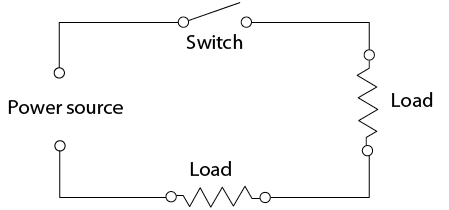Skip to main content

# 2.2: Series Circuits

•• Contributed by Camosun College
• Sourced from BCCampus (Download for free at http://open.bccampus.ca/find-open-textbooks)

Series circuits

The electrical term in series refers to a circuit in which two or more components are connected one after another in order that the current can only flow through one path. The switch that controls the circuit is always in series with the loads. If more than one switch is used, both must be closed for the circuit to function. Circuit protectors (such as fuses) will also be in series. If any one of the components in a series circuit opens, the circuit will not function (Figure 3).Figure $$\PageIndex{1}$$: (CC BY-NC-SA; BC Industry Training Authority)
1. Series circuitFigure $$\PageIndex{1}$$: (CC BY-NC-SA; BC Industry Training Authority)
2. Series circuit

Perform the following calculations:

1. Total resistance or RT will equal the sum of the individual resistances.

RT = R1 + R2 + R3

RT = 2 Ω + 4 Ω + 6 Ω

RT = 12 Ω

2. Now apply Ohm’s law and calculate the current flow in the circuit.

I = E ÷ R

I = 12 V ÷ 12 Ω

I = 1 A

3. Current flow is the same throughout the circuit. By using Ohm’s law you can determine how much voltage will be used by each of the loads.

The 2 ohm resistor will require:

E = I × R

E = 1 A × 2 Ω

E = 2 V

The 4 ohm resistor will require:

E = I × R

E = 1 A × 4 Ω

E = 4 V

The 6 ohm resistor will require:

E = I × R

E = 1 A × 6 Ω

E = 6 V

Add the individual voltages together and you will notice that they equal the original source voltage of 12 V.

ET = 2 V + 4 V + 6 V

ET = 12 V

The voltage that is used up in the circuit by the load is called voltage drop. This voltage drop is valuable in diagnosis as a measure of the resistance of a circuit. Some voltage may be lost in a circuit because of poor connections. If the voltage drop in connections (caused by high resistance) becomes too great, the load may not function properly or may not even work.